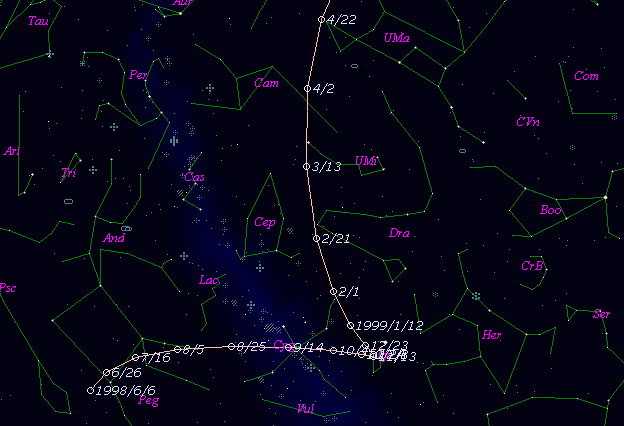# \$B%j%K%"WB@1(B

C/1998 M5 ( LINEAR )

 English version Home page Updated on December 31, 2016###\$B%W%m%U%#!<%k(B

 \$BH/8+F|(B 1998\$BG/(B6\$B7n(B30\$BF|(B \$BH/8+8wEY(B 15.9\$BEy(B \$BH/8+ Lincoln Laboratory Near-Earth Asteroid Research project

###\$B###\$B50F;MWAG(B

```   The following improved orbital elements by Kenji Muraoka, are
from 1150 observations 1998 June 30 to 1999 Dec. 27, perturbations
by 8 Planets, Moon and 3 minor planets were taken into account.
The mean residual is +/- 0.64 arc seconds.

Epoch  =  1999 Jan  = 2451200.5
T  =  1999 Jan. 24.57126       +/- 0.00014 (m.e.) TT
Peri. =  101.28447                +/- 0.00005
Node  =  333.37676                +/- 0.00003   (2000.0)
Incl. =   82.22886                +/- 0.00004
q  =    1.7422802              +/- 0.0000009 AU
e  =    0.9960389              +/- 0.0000025
1/a  =   +0.0022735              +/- 0.0000014 1/AU
( P  =   9225 years )
orig. 1/a  =   +0.0005144
fut.  1/a  =   +0.0026141
```

###\$B@1?^(B###\$B8wEYJQ2=(B

```        m1 = 6.0 + 5 log\$B&\$(B + 10.0 log r
```##### \$B50F;MWAG\$OB<2,7r<#;a\$N7W;;\$K\$h\$k\$b\$N\$G\$9!#(B \$B@1?^\$O(B StellaNavigator Ver.2.0 for Windows (\$B%"%9%H%m%"!<%D(B \$BJTCx(B / \$B%"%9%-!<=PHG6I4)(B) \$B\$G:n@.\$7\$?\$b\$N\$G\$9!#(B \$B8wEY%0%i%U\$O(BComet for Windows\$B\$G:n@.\$7\$?\$b\$N\$G\$9!#(B